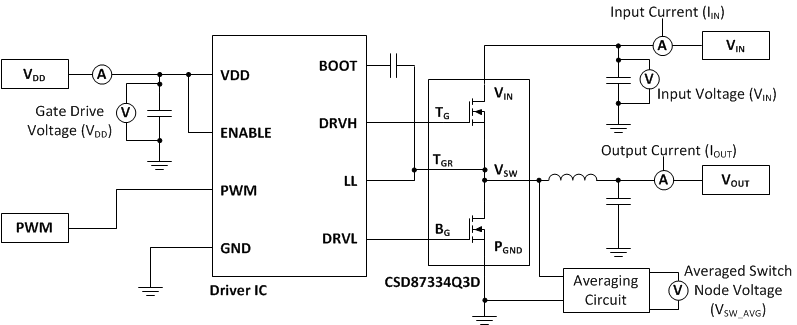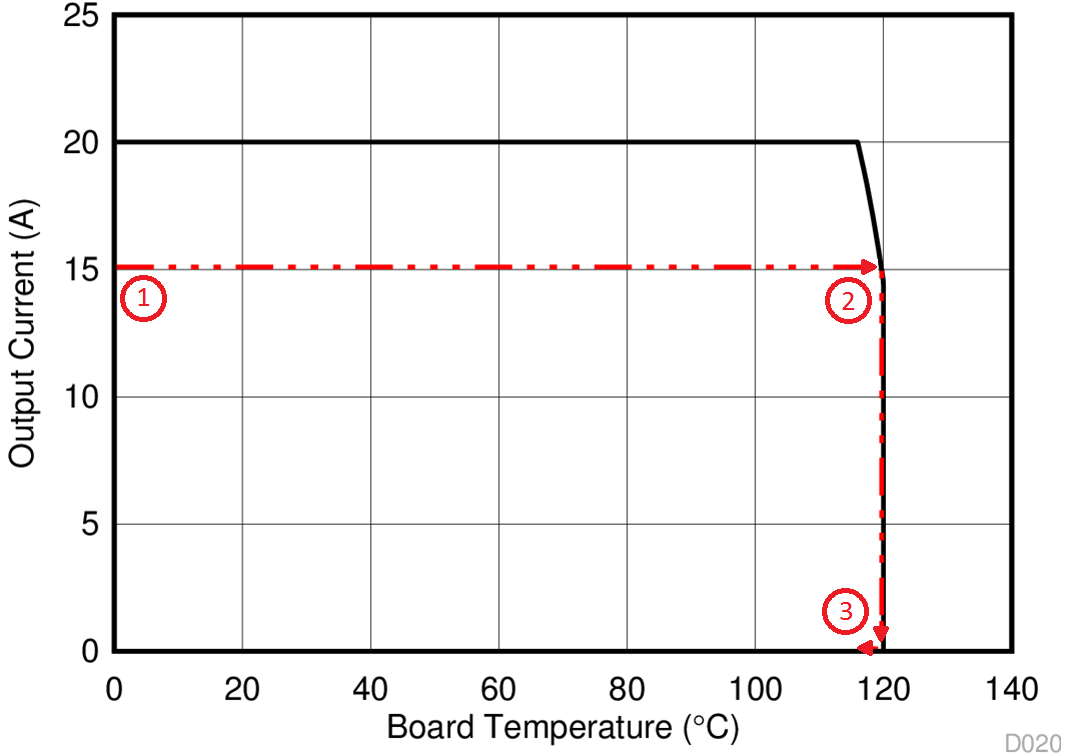SLPS546A July   2015  – March 2017

PRODUCTION DATA.

1. 1Features
2. 2Applications
3. 3Description
4. 4Revision History
5. 5Specifications
6. 6Application and Implementation
1. 6.1 Application Information
2. 6.2 Typical Application
3. 6.3 System Example
7. 7Layout
1. 7.1 Layout Guidelines
2. 7.2 Layout Example
3. 7.3 Thermal Considerations
8. 8Device and Documentation Support
9. 9Mechanical, Packaging, and Orderable Information

• DPB|8

## 6 Application and Implementation

NOTE

Information in the following applications sections is not part of the TI component specification, and TI does not warrant its accuracy or completeness. TI’s customers are responsible for determining suitability of components for their purposes. Customers should validate and test their design implementation to confirm system functionality.

### 6.1 Application Information

The CSD87334Q3D NexFET power block is an optimized design for synchronous buck applications using 5-V gate drive. The control FET and sync FET silicon are parametrically tuned to yield the lowest power loss and highest system efficiency. As a result, a new rating method is needed which is tailored towards a more systems-centric environment. System-level performance curves such as power loss, Safe Operating Area, and normalized graphs allow engineers to predict the product performance in the actual application.

### 6.2 Typical ApplicationFigure 19. Typical Circuit Application

### 6.3 System Example

#### 6.3.1 Power Loss Curves

MOSFET centric parameters such as RDS(ON) and Qgd are needed to estimate the loss generated by the devices. In an effort to simplify the design process for engineers, Texas Instruments has provided measured power loss performance curves. Figure 1 plots the power loss of the CSD87334Q3D as a function of load current. This curve is measured by configuring and running the CSD87334Q3D as it would be in the final application (see Figure 19). The measured power loss is the CSD87334Q3D loss and consists of both input conversion loss and gate drive loss. Equation 1 is used to generate the power loss curve.

Equation 1. Power loss = (VIN × IIN) + (VDD × IDD) – (VSW_AVG × IOUT)

The power loss curve in Figure 1 is measured at the maximum recommended junction temperatures of 125°C under isothermal test conditions.

#### 6.3.2 Safe Operating Area (SOA) Curves

The SOA curves in the CSD87334Q3D data sheet provides guidance on the temperature boundaries within an operating system by incorporating the thermal resistance and system power loss. Figure 3 to Figure 5 outline the temperature and airflow conditions required for a given load current. The area under the curve dictates the SOA. All the curves are based on measurements made on a PCB design with dimensions of 4 in (W) × 3.5 in (L) × 0.062 in (T) and 6 copper layers of 1-oz copper thickness.

#### 6.3.3 Normalized Curves

The normalized curves in the CSD87334Q3D data sheet provides guidance on the power loss and SOA adjustments based on their application specific needs. These curves show how the power loss and SOA boundaries adjust for a given set of system conditions. The primary Y-axis is the normalized change in power loss, and the secondary Y-axis is the change is system temperature required in order to comply with the SOA curve. The change in power loss is a multiplier for the power loss curve and the change in temperature is subtracted from the SOA curve.

#### 6.3.4 Calculating Power Loss and SOA

The user can estimate product loss and SOA boundaries by arithmetic means (see Design Example section). Though the power loss and SOA curves in this data sheet are taken for a specific set of test conditions, the following procedure outlines the steps the user should take to predict product performance for any set of system conditions.

#### 6.3.4.1 Design Example

Operating conditions:

• Output current = 15 A
• Input voltage = 16 V
• Output voltage = 5 V
• Switching frequency = 1000 kHz
• Inductor = 0.6 µH

#### 6.3.4.2 Calculating Power Loss

• Power loss at 15 A = 2.8 W (Figure 1)
• Normalized power loss for input voltage ≈ 1.05 (Figure 7)
• Normalized power loss for output voltage ≈ 1.08 (Figure 8)
• Normalized power loss for switching frequency ≈ 1.03 (Figure 6)
• Normalized power loss for output inductor ≈ 1.05 (Figure 9)
• Final calculated power loss = 2.8 W × 1.05 × 1.08 × 1.03 × 1.05 ≈ 3.4 W

• SOA adjustment for input voltage ≈ 0.5°C (Figure 7)
• SOA adjustment for output voltage ≈ 0.7°C (Figure 8)
• SOA adjustment for switching frequency ≈ 0.3°C (Figure 6)
• SOA adjustment for output inductor ≈ 0.5°C (Figure 9)
• Final calculated SOA adjustment = 0.5 + 0.7 + 0.3 + 0.5 ≈ 2°C

In the design example, the estimated power loss of the CSD87334Q3D would increase to 3.4 W. In addition, the maximum allowable board or ambient temperature, or both, would have to decrease by 2°C. Figure 20 graphically shows how the SOA curve would be adjusted accordingly.

1. Start by drawing a horizontal line from the application current to the SOA curve.
2. Draw a vertical line from the SOA curve intercept down to the board or ambient temperature.
3. Adjust the SOA board or ambient temperature by subtracting the temperature adjustment value.

In the design example, the SOA temperature adjustment yields a reduction in allowable board/ambient temperature of 2°C. In the event the adjustment value is a negative number, subtracting the negative number would yield an increase in allowable board or ambient temperature.

.Figure 20. Power Block SOA